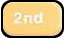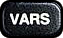Tech Tips: Poisson Distributions

Calculating $P(X=x)$ when $X$ follows a Poisson Distribution

Suppose one wishes to find the Poisson probability of seeing exactly $k$ occurrences of some event within some well-defined interval, where the mean number of occurrences in that interval is expected to be $\lambda$. That is to say, we seek

$$P(k) = \frac{e^{-\lambda} \lambda^k}{k!}$$

To do this one should ...

• R: use the function

dpois(x=k, lambda=λ)

As an example, the probability of seeing exactly 3 blemishes on a randomly selected piece of sheet metal, when on average one expects 1.2 blemishes, can be found with::

> dpois(x=3, lambda=1.2)
 0.2734375

• Excel: use the function

POISSON.DIST(k, λ, FALSE)

The last argument for this function, when $FALSE$, indicates that the probability returned should not be cumulative (i.e., it only returns $P(k)$, not $P(0) + P(1) + \cdots + P(k)$).

• TI-83: use the function

poissonpdf(λ,k)

This function can be found by making the following menu selections:: poissonpdf(

Calculating Cumulative Probabilities when $X$ follows a Poisson Distribution

Suppose one wishes to fine the cumulative Poisson probability of seeing $k$ or fewer occurrences of some event within some well-defined interval or range, where the mean number of occurrences in that interval is expected to be $\lambda$. That is to say, we seek $$P(X \le k) = P(0) + P(1) + P(2) + \cdots + P(k) = \sum_{0 \le i \le k} \frac{e^{-\lambda} \lambda^k}{k!}$$ To do this, one should ...

• R: use the function

ppois(x=k, lambda=λ)

As an example, suppose that in a given call center that gets on average 13 calls every hour, one can calculate the probability that in a given $15$ minute period the call center will receive less than $6$ calls with the following:

> ppois(5, lambda = 13/4)
 0.8888132

• Excel: use the function

POISSON.DIST(k, λ, TRUE)

The last argument for this function, when $TRUE$, indicates the probability returned should be cumulative. That is to say, it gives the sum $P(0) + P(1) + \cdots + P(k)$.

• TI-83: use the function

poissoncdf(λ,k)

This function can be found by making the following menu selections:: poissoncdf(

Simulating Random Variables following Poisson Distributions

To generate $n$ realizations of a random variable that follows a Poisson distribution, counting the number of occurrences of some event within some well-defined interval or range, where the mean number of occurrences in that interval is expected to be $\lambda$. ...
• R: use the function

rpois(x=n, lambda=λ)

As an example, suppose over the course of 15 weeks, every Saturday -- at the same time -- an individual stands by the side of a road and tallies the number of cars going by within a 120-minute window. To simulate a set of observations for this situation under the assumption that the mean number of cars that go by per hour is actually $52$, one can use:

> rpois(15, lambda=104)
  96 113 106 104 101  96  96 114 109  91  94  95 108 113 113Donald Knuth For the curious, there is also a simple algorithm (in the sense of only using basic math functions) for generating Poisson-distributed numbers, attributed to Donald Knuth. The R implementation of this algorithm is shown below.

generate.poisson.value = function(lambda) {
L = exp(-lambda)
k = 0
p = 1
repeat {
k = k + 1
p = p * runif(1)
if (p <= L) {
break
}
}
return(k-1)
}

• Excel: There is no built-in Poisson analog to BINOM.INV(), so Poisson-distributed numbers can't be generated in the same way. If one absolutely has to generate Poisson-distributed numbers in Excel, one should look up how to create a VBA (i.e., Visual Basic) script to execute Donald Knuth's algorithm described above.